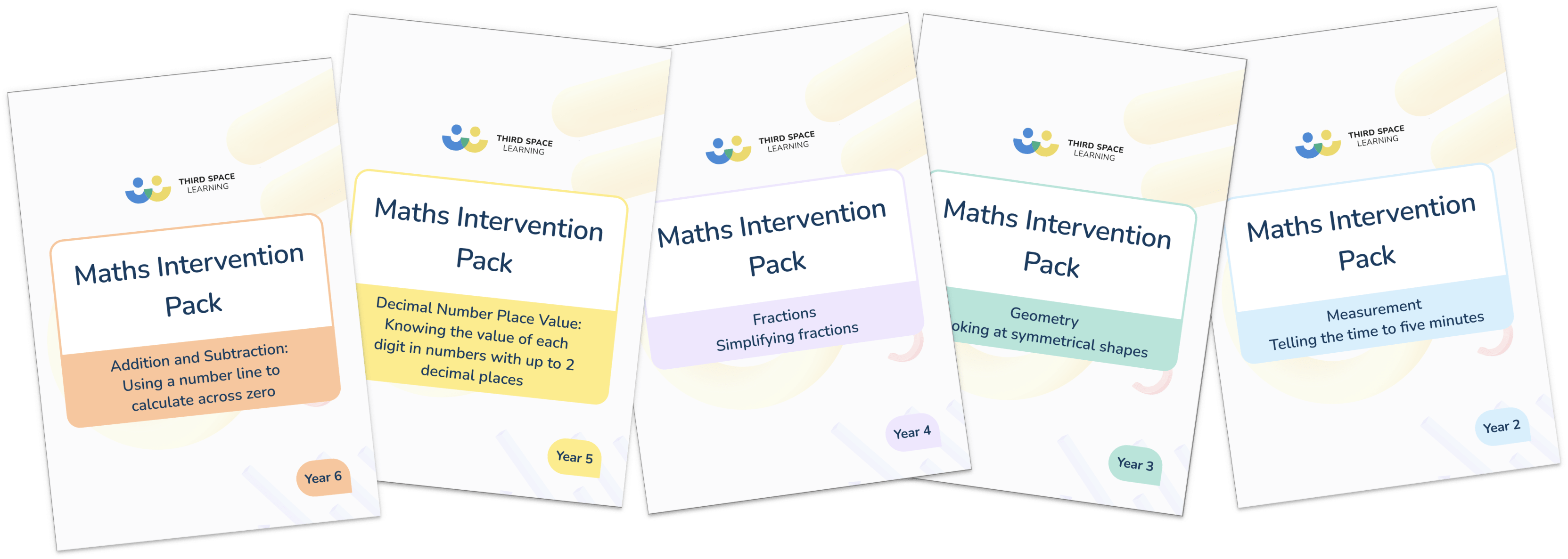# Ultimate Pack Of Maths Intervention Lessons60+ intervention lessons ready for you to use one to one or in small groups (Year 2, 3, 4, 5, 6).

• Place value (10 lessons)
• Addition and subtraction (10 lessons)
• Multiplication and division (9 lessons)
• Fractions (9 lessons)
• Geometry (9 lessons)
• Measurement (9 lessons)
• Decimal place value (5 lessons)
• Includes notes, answers and slides
• As used in the Third Space Learning tutoring programmes